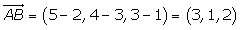Learn from home

The teachersChapters

Three or more points are collinear if they are part of the same straight line and as a result the rank of the vectors is 1.

1.Determine if the points A = (2, 3, 1), B = (5, 4, 3) and C = (2, 1, 2) are collinear.The points are not collinear.

## Midpoint

If A = (x1, y1, z1) and B = (x2, y2, z2) are the endpoints of a line segment, the midpoint of the line segment is given by:1.Given the endpoints A = (3, −2, 5) and B = (3, 1, 7), find the coordinates of the midpoint of the line segment that they determine.2.The coordinates of consecutive vertices of a parallelogram are A = (1, 0, 0) and B = (0, 1, 0). If the center coordinates of M are (0, 0, 1), find the coordinates of the vertices C and D.Did you like the article?(1 votes, average: 5.00 out of 5)Loading...

Emma

I am passionate about travelling and currently live and work in Paris. I like to spend my time reading, gardening, running, learning languages and exploring new places.

Did you like
this resource?

Bravo!